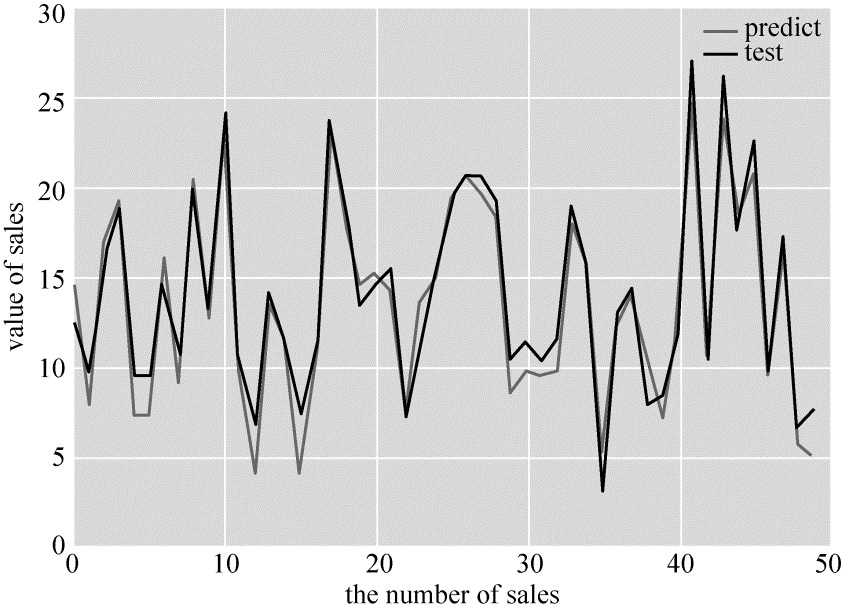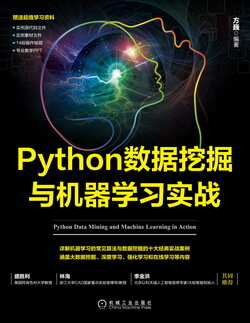# Python 数据挖掘与机器学习实战 (63)：回归分析介 3.4.3(线性回归模型)

• 优点：快速；没有调节参数；可轻易解释；可理解。
• 缺点：相比其他复杂一些的模型，其预测准确率不高，因为它假设特征和响应之间存在确定的线性关系，这种假设对于非线性的关系，线性回归模型显然不能很好地进行数据建模。

1．使用 pandas 构建 X（特征向量）和 y（标签列）

scikit-learn 要求 $X$$X$ 是一个特征矩阵，$y$$y$ 是一个 NumPy 向量。pandas 构建在 NumPy 之上。因此，$X$$X$ 可以是 pandas 的 DataFrame，$y$$y$ 可以是 pandas 的 Series，scikit-learn 可以理解这种结构。

#创建特征列表feature_cols = ['TV', 'Radio', 'Newspaper']#使用列表选择原始 DataFrame 的子集X = data[feature_cols]X = data[['TV', 'Radio', 'Newspaper']]# 输出前 5 项数据print (X.head())

print (type(X))print (X.shape)

      TV      Radio    Newspaper0    230.1     37.8       69.21    44.5      39.3       45.12    17.2      45.9       69.33    151.5     41.3       58.54    180.8     10.8       58.4<class 'pandas.core.frame.DataFrame'>(200, 3)

#从 DataFrame 中选择一个 Seriesy = data['Sales']y = data.Sales#输出前 5 项数据print (y.head())

0    22.11    10.42     9.33    18.54    12.9Name: Sales

2．构建训练集与测试集

<pre name="code" class="python"><span style="font-size:14px;">##构造训练    集和测试集from sklearn.cross_validation import train_test_split   #这里是引用交叉验证X_train,X_test, y_train, y_test = train_test_split(X, y, random_state=1)# 75% 用于训练,25% 用于测试print (X_train.shape)print (y_train.shape)print (X_test.shape)print (y_test.shape)

(150,3)(150,)(50,3)(50,)

3．sklearn 的线性回归

from sklearn.linear_model import LinearRegressionlinreg = LinearRegression()model=linreg.fit(X_train, y_train)              #线性回归print (model)print (linreg.intercept_)           #输出结果print (linreg.coef_)

LinearRegression(copy_X=True, fit_intercept=True, normalize=False)2.66816623043[ 0.04641001  0.19272538 -0.00349015]

# 将特征名称与系数对应zip(feature_cols, linreg.coef_)

[('TV', 0.046410010869663267), ('Radio', 0.19272538367491721), ('Newspaper', -0.0034901506098328305)]

$y=2.66+0.0464×TV+0.192×Radio-0.00349×Newspaper\phantom{\rule{1em}{0ex}}\phantom{\rule{1em}{0ex}}\phantom{\rule{1em}{0ex}}\phantom{\rule{1em}{0ex}}\phantom{\rule{1em}{0ex}}\phantom{\rule{1em}{0ex}}\phantom{\rule{1em}{0ex}}\left(3-8\right)$$y=2.66+0.0464\times TV+0.192\times Radio-0.00349\times Newspaper\quad\quad\quad\quad\quad\quad\quad(3-8)$

4．预测

y_pred = linreg.predict(X_test)print (y_pred)print (type(y_pred))

[ 14.58678373   7.92397999   16.9497993   19.35791038  7.36360284  7.35359269   16.08342325  9.16533046   20.35507374  12.63160058  22.83356472  9.66291461   4.18055603   13.70368584  11.4533557  4.16940565   10.31271413  23.06786868  17.80464565  14.53070132  15.19656684  14.22969609  7.54691167   13.47210324  15.00625898  19.28532444  20.7319878   19.70408833  18.21640853  8.50112687  9.8493781    9.51425763   9.73270043   18.13782015  15.41731544  5.07416787   12.20575251  14.05507493  10.6699926   7.16006245  11.80728836  24.79748121  10.40809168  24.05228404  18.44737314  20.80572631  9.45424805   17.00481708  5.78634105   5.10594849]<type 'numpy.ndarray'>

5．评价测度

• 平均绝对误差（Mean Absolute Error，MAE）；
• 均方误差（Mean Squared Error，MSE）；
• 均方根误差（Root Mean Squared Error，RMSE）。

#计算 Sales 预测的 RMSEprint (type(y_pred),type(y_test))print (len(y_pred),len(y_test))print (y_pred.shape,y_test.shape)from sklearn import metricsimport numpy as npsum_mean=0for i in range(len(y_pred)):    sum_mean+=(y_pred[i]-y_test.values[i])**2sum_erro=np.sqrt(sum_mean/50)# 计算 RMSE 的大小print ("RMSE by hand:",sum_erro)

<type 'numpy.ndarray'><class 'pandas.core.series.Series'>50 50(50,) (50,)RMSE by hand: 1.42998147691

import matplotlib.pyplot as pltplt.figure()plt.plot(range(len(y_pred)),y_pred,'b',label="predict")plt.plot(range(len(y_pred)),y_test,'r',label="test")plt.legend(loc="upper right")               #显示图中的标签plt.xlabel("the number of sales")             #横坐标轴plt.ylabel('value of sales')                  #纵坐标轴plt.show()#显示结果